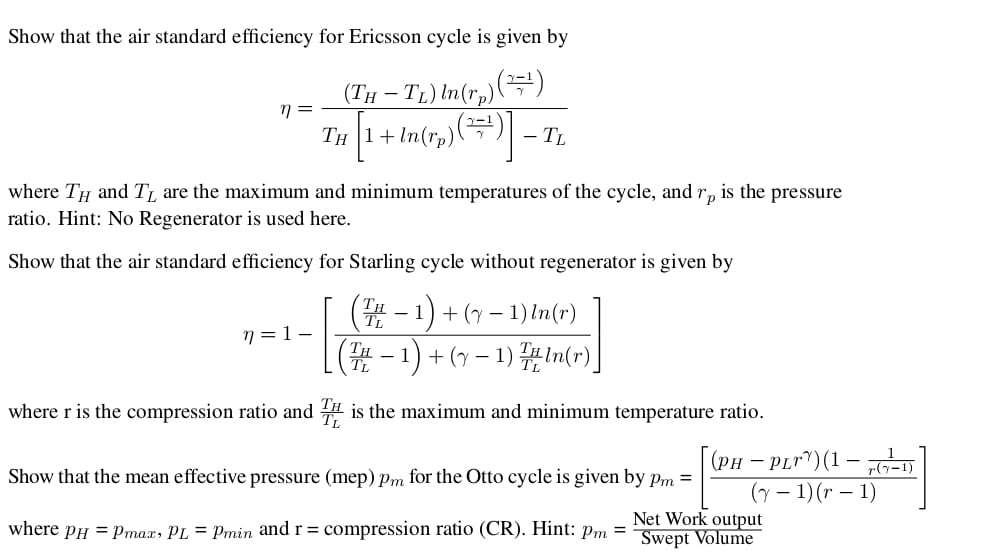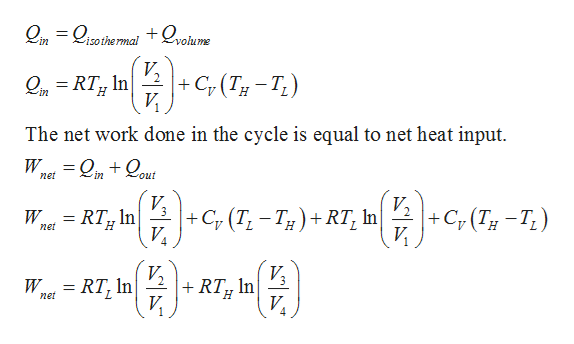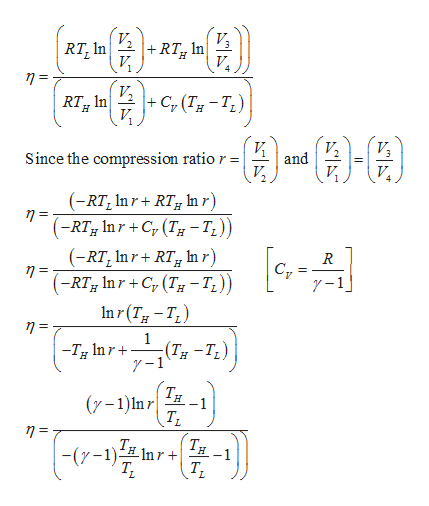# Show that the air standard efficiency for Ericsson cycle is given by-T) In(r,)()Тн 1+ In(rp)((ТH- TLwhere TH and T, are the maximum and minimum temperatures of the cycle, andis the pressureГрratio. Hint: No Regenerator is used here.Show that the air standard efficiency for Starling cycle without regenerator is given by(-1)+-1) n(r)(T-1)1)n(r)n=1 -where r is the compression ratio and is the maximum and minimum temperature ratio.(рн — PLr)(1 — -DShow that the mean effective pressure (mep) pm for the Otto cycle is given by pm =(-1)(r - 1)Net Work outputSwept Volumewhere pH max, PL = Pmin and r = compression ratio (CR). Hint: pm

Question
191 views

take your time but please solve the question related to starling cycle and otto cycle.

they are the subparts of the same question.

try sending solution in 2 hours atleast if possible.help_outlineImage TranscriptioncloseShow that the air standard efficiency for Ericsson cycle is given by -T) In(r,)() Тн 1+ In(rp)( (ТH - TL where TH and T, are the maximum and minimum temperatures of the cycle, and is the pressure Гр ratio. Hint: No Regenerator is used here. Show that the air standard efficiency for Starling cycle without regenerator is given by (-1)+-1) n(r) (T-1)1)n(r) n=1 - where r is the compression ratio and is the maximum and minimum temperature ratio. (рн — PLr)(1 — -D Show that the mean effective pressure (mep) pm for the Otto cycle is given by pm = (-1)(r - 1) Net Work output Swept Volume where pH max, PL = Pmin and r = compression ratio (CR). Hint: pm fullscreen
check_circle

Step 1

Hello. Since you have posted multiple questions which are needed to be solved, we will solve the first question for you. If you want any other specific question to be solved then please resubmit only that question or specify the question number.

Consider the P-V and T-S diagram of the Stirling cycle.

The description of the processes are as follows,

Process 1-2 – Isothermal heat rejection.

Process 2-3 – Constant volume heat addition.

Process 3-4 – Isothermal heat addition. Volume of the system increases due to isothermal heat addition.

Process 4-1 – Constant volume heat rejection. Both temperature and pressure of the system decreases due to isochoric heat removal.

Step 2

The net heat input in the Stirling cycle is equal to the heat addition in isothermal process and heat addition in constant volume process.help_outlineImage Transcriptionclose= Qisothe rmal+Qyokume in V |+ C,(T-T;) In OiRTH V The net work done in the cycle is equal to net heat input W out in net V2 V. +Cy (T-T) |+ Cf (T,-T)+RT, In V W net = RT, In| = RT, In V In V W net fullscreen
Step 3

Write the expression for the a...help_outlineImage TranscriptioncloseV. |+ RT RT, In VL 4 n = Cy (7-T) RTH In Since the compression ratio r = and V (RT, In r RT Inr) (-RTg Inr+C (T-T,)) (-RT, Inr RT In r) (-RT;g Inr +C,(T -T,)) Inr(TT R 1-1 1 (T-T 7-1 -T Inr H (y-1)In r -1 -(y-1)n --1 fullscreen

### Want to see the full answer?

See Solution

#### Want to see this answer and more?

Solutions are written by subject experts who are available 24/7. Questions are typically answered within 1 hour.*

See Solution
*Response times may vary by subject and question.
Tagged in

### Heat Transfer